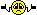1. ## LX10 resistor question

I just finished replacing the 12uf caps in a pair of LX10 crossovers. In the process I noticed that the value of R1 as installed is 4 ohms. Looking at the schematic of the LX10, the indicated value of R1 is 5.1ohms. One of the notes on the drawing seems to indicate that R1 was revised and the drawing was redrawn. The dates are a bit hard to read but I think they are '77 or '80.

The crossovers I have came out of a set of C56 Dorians with S1 system, probably from the late '60s. I'm wondering if R1 was revised from 4ohm to 5.1ohm when this drawing was redrawn? If so, should I change the 4ohm resistors to 5.1? These have the old white LE14's. I'm thinking maybe the revision was made when the LE14 went from the Aquaplas cone to the paper, or wouldn't that have anything to do with the resistor?

On another note, the value of R2 should be 7.5ohms. One of the xovers has a 7.5ohm resistor placed just as shown in the drawing. The other has a 15ohm resistor in parallel with a third resistor which is also 15ohm but only 5w as opposed to 10w like the other 15 ohm resistor is. The net electrical result is the same as a single 7.5ohm, bit I'm curious as to why JBL would do this? The serial #s are only about 100 apart.

http://www.jblproservice.com/pdf/Net...atics/LX10.pdfReply With Quote

2.Originally Posted by jimbofishOn another note, the value of R2 should be 7.5ohms. One of the xovers has a 7.5ohm resistor placed just as shown in the drawing. The other has a 15ohm resistor in parallel with a third resistor which is also 15ohm but only 5w as opposed to 10w like the other 15 ohm resistor is. The net electrical result is the same as a single 7.5ohm, bit I'm curious as to why JBL would do this? The serial #s are only about 100 apart.

http://www.jblproservice.com/pdf/Net...atics/LX10.pdf
Maybe the 7.5 ohms 10W were out of stock or more expensive than two 15 ohms 5WReply With Quote

3. The resistors form a fixed L-Pad attenuator, which sets an upper limit to the amount of power that is sent to the high frequency driver. And this also reduces the heat load on the variable 8 ohm L-Pad network. A 4 ohm series resistor combined with a 7.5 ohm shunt resistor will drop to signal by 6.2 db. While a 5.1 ohm series resistor combined with a 7.5 ohm shunt resistor will drop to signal by 7.3 db. The difference of 1.1 db can be easily be balanced out by the variable L-Pad network. But, the impedance load on the C1 and L1 high pass network is different, meaning that the crossover point would be shifted slightly between the two networks. And I am not sure that this would be noticeable as the impedance would be 7.87 ohms with the 4 ohms series resistor and 8.97 ohms with the 5.1 ohm series resistor. Still, if these were my crossover networks, I would want them to match. So, I would suggest replacing the 4 ohm R1 resistor with a 5.1 ohm resistor.
Baron030Reply With Quote

4.Originally Posted by EauliveMaybe the 7.5 ohms 10W were out of stock or more expensive than two 15 ohms 5WOriginally Posted by Baron030The resistors form a fixed L-Pad attenuator, which sets an upper limit to the amount of power that is sent to the high frequency driver. And this also reduces the heat load on the variable 8 ohm L-Pad network. A 4 ohm series resistor combined with a 7.5 ohm shunt resistor will drop to signal by 6.2 db. While a 5.1 ohm series resistor combined with a 7.5 ohm shunt resistor will drop to signal by 7.3 db. The difference of 1.1 db can be easily be balanced out by the variable L-Pad network. But, the impedance load on the C1 and L1 high pass network is different, meaning that the crossover point would be shifted slightly between the two networks. And I am not sure that this would be noticeable as the impedance would be 7.87 ohms with the 4 ohms series resistor and 8.97 ohms with the 5.1 ohm series resistor. Still, if these were my crossover networks, I would want them to match. So, I would suggest replacing the 4 ohm R1 resistor with a 5.1 ohm resistor.
Baron030Maybe I didn't explain clearly. It's the drawing of the schematic that specifies it as 5.1. Both of the xovers currently have the same 4ohm resistor installed, so they are both identical... except for the 2 15ohm resistors in parallel on one. I would expect them to work the same, no?

So, what would the difference in crossover points be between the 4 and 5.1 ohm system?Reply With Quote

5. Ok, if both crossover networks have 4 ohm resistors for the R1 value, then you dont need to change them. And since, two 15 ohms resistor in parallel is equal to a single 7.5 ohm resistor then there is no need to change those resistors either. I am away from any crossover network software right now, so I cant do any calculations. But, off the top of my head, switching the R1 values to 5.1 ohms would actually lower the crossover point on the high frequency driver by a very small amount. And I suspect that the difference would not be very noticable.
Baron030Reply With Quote

6. Well, I don't see any reason to change them out then.

I still wonder why they made the change... or if there are earlier drawings that spec 4 ohm resistors?Reply With Quote